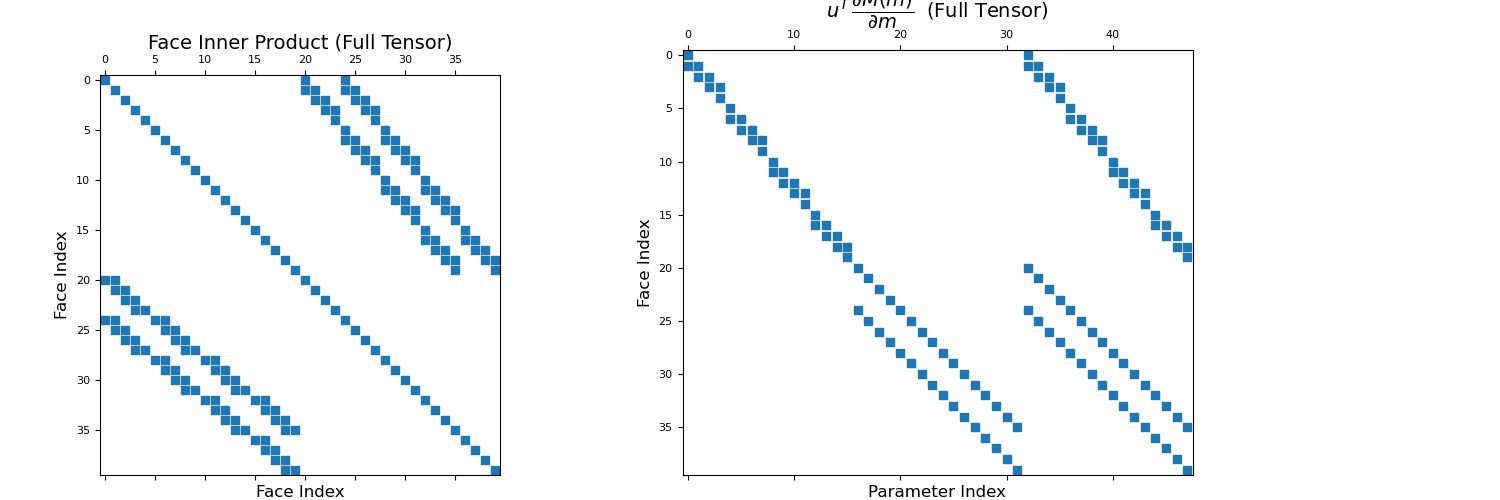# discretize.CylindricalMesh.get_face_inner_product_deriv¶

CylindricalMesh.get_face_inner_product_deriv(model, do_fast=True, invert_model=False, invert_matrix=False, **kwargs)[source]

Function handle to multiply vector with derivative of face inner product matrix (or its inverse).

Let $$\mathbf{M}(\mathbf{m})$$ be the face inner product matrix constructed with a set of physical property parameters $$\mathbf{m}$$ (or its inverse). get_face_inner_product_deriv constructs a function handle

$\mathbf{F}(\mathbf{u}) = \mathbf{u}^T \, \frac{\partial \mathbf{M}(\mathbf{m})}{\partial \mathbf{m}}$

which accepts any numpy.array $$\mathbf{u}$$ of shape (n_faces,). That is, get_face_inner_product_deriv constructs a function handle for computing the dot product between a vector $$\mathbf{u}$$ and the derivative of the face inner product matrix (or its inverse) with respect to the property parameters. When computed, $$\mathbf{F}(\mathbf{u})$$ returns a scipy.sparse.csr_matrix of shape (n_faces, n_param).

The function handle can be created for isotropic, diagonal isotropic and full tensor physical properties; see notes.

Parameters
modelnumpy.ndarray

Parameters defining the material properties for every cell in the mesh. Inner product matrices can be constructed for the following cases:

• (n_cells) numpy.ndarray : Isotropic case. model contains a scalar physical property value for each cell.

• (n_cells, dim) numpy.ndarray : Diagonal anisotropic case. Columns are ordered np.c_[σ_xx, σ_yy, σ_zz]. This can also a be a 1D array with the same number of total elements in column major order.

• (n_cells, 3) numpy.ndarray (dim is 2) or (n_cells, 6) numpy.ndarray (dim is 3) : Full tensor properties case. Columns are ordered np.c_[σ_xx, σ_yy, σ_zz, σ_xy, σ_xz, σ_yz] This can also be a 1D array with the same number of total elements in column major order.

invert_modelbool, optional

The inverse of model is used as the physical property.

invert_matrixbool, optional

Returns the inverse of the inner product matrix. The inverse not implemented for full tensor properties.

do_fastbool, optional

Do a faster implementation (if available).

Returns
function

The function handle $$\mathbf{F}(\mathbf{u})$$ which accepts a (n_faces) numpy.ndarray $$\mathbf{u}$$. The function returns a (n_faces, n_params) scipy.sparse.csr_matrix.

Notes

Let $$\mathbf{M}(\mathbf{m})$$ be the face inner product matrix (or its inverse) for the set of physical property parameters $$\mathbf{m}$$. And let $$\mathbf{u}$$ be a discrete quantity that lives on the faces. get_face_inner_product_deriv creates a function handle for computing the following:

$\mathbf{F}(\mathbf{u}) = \mathbf{u}^T \, \frac{\partial \mathbf{M}(\mathbf{m})}{\partial \mathbf{m}}$

The dimensions of the sparse matrix constructed by computing $$\mathbf{F}(\mathbf{u})$$ for some $$\mathbf{u}$$ depends on the constitutive relation defined for each cell. These cases are summarized below.

Isotropic Case: The physical property for each cell is defined by a scalar value. Therefore $$\mathbf{m}$$ is a (n_cells) numpy.ndarray. The sparse matrix output by computing $$\mathbf{F}(\mathbf{u})$$ has shape (n_faces, n_cells).

Diagonal Anisotropic Case: In this case, the physical properties for each cell are defined by a diagonal tensor

$\begin{split}\Sigma = \begin{bmatrix} \sigma_{xx} & 0 & 0 \\ 0 & \sigma_{yy} & 0 \\ 0 & 0 & \sigma_{zz} \end{bmatrix}\end{split}$

Thus there are dim * n_cells physical property parameters and $$\mathbf{m}$$ is a (dim * n_cells) numpy.ndarray. The sparse matrix output by computing $$\mathbf{F}(\mathbf{u})$$ has shape (n_faces, dim * n_cells).

Full Tensor Case: In this case, the physical properties for each cell are defined by a full tensor

$\begin{split}\Sigma = \begin{bmatrix} \sigma_{xx} & \sigma_{xy} & \sigma_{xz} \\ \sigma_{xy} & \sigma_{yy} & \sigma_{yz} \\ \sigma_{xz} & \sigma_{yz} & \sigma_{zz} \end{bmatrix}\end{split}$

Thus there are 6 * n_cells physical property parameters in 3 dimensions, or 3 * n_cells physical property parameters in 2 dimensions, and $$\mathbf{m}$$ is a (n_params) numpy.ndarray. The sparse matrix output by computing $$\mathbf{F}(\mathbf{u})$$ has shape (n_faces, n_params).

Examples

Here, we construct a 4 cell by 4 cell tensor mesh. For our first example we consider isotropic physical properties; that is, the physical properties of each cell are defined a scalar value. We construct the face inner product matrix and visualize it with a spy plot. We then use get_face_inner_product_deriv to construct the function handle $$\mathbf{F}(\mathbf{u})$$ and plot the evaluation of this function on a spy plot.

>>> from discretize import TensorMesh
>>> import matplotlib.pyplot as plt
>>> import numpy as np
>>> import matplotlib as mpl
>>> mpl.rcParams.update({'font.size': 14})
>>> np.random.seed(45)
>>> mesh = TensorMesh([[(1, 4)], [(1, 4)]])


Define a model, and a random vector to multiply the derivative with, then we grab the respective derivative function and calculate the sparse matrix,

>>> m = np.random.rand(mesh.nC)  # physical property parameters
>>> u = np.random.rand(mesh.nF)  # vector of shape (n_faces)
>>> Mf = mesh.get_face_inner_product(m)
>>> F = mesh.get_face_inner_product_deriv(m)  # Function handle
>>> dFdm_u = F(u)


Spy plot for the inner product matrix and its derivative

Expand to show scripting for plot
>>> fig = plt.figure(figsize=(15, 5))
>>> ax1 = fig.add_axes([0.05, 0.05, 0.3, 0.85])
>>> ax1.spy(Mf, ms=6)
>>> ax1.set_title("Face Inner Product Matrix (Isotropic)", fontsize=14, pad=5)
>>> ax1.set_xlabel("Face Index", fontsize=12)
>>> ax1.set_ylabel("Face Index", fontsize=12)
>>> ax2 = fig.add_axes([0.43, 0.05, 0.17, 0.8])
>>> ax2.spy(dFdm_u, ms=6)
>>> ax2.set_title(
...     "$u^T \, \dfrac{\partial M(m)}{\partial m}$ (Isotropic)",
... )
>>> ax2.set_xlabel("Parameter Index", fontsize=12)
>>> ax2.set_ylabel("Face Index", fontsize=12)
>>> plt.show()For our second example, the physical properties on the mesh are fully anisotropic; that is, the physical properties of each cell are defined by a tensor with parameters $$\sigma_1$$, $$\sigma_2$$ and $$\sigma_3$$. Once again we construct the face inner product matrix and visualize it with a spy plot. We then use get_face_inner_product_deriv to construct the function handle $$\mathbf{F}(\mathbf{u})$$ and plot the evaluation of this function on a spy plot.

>>> m = np.random.rand(mesh.nC, 3)  # anisotropic physical property parameters
>>> u = np.random.rand(mesh.nF)     # vector of shape (n_faces)
>>> Mf = mesh.get_face_inner_product(m)
>>> F = mesh.get_face_inner_product_deriv(m)  # Function handle
>>> dFdm_u = F(u)


Plot the anisotropic inner product matrix and its derivative matrix,

Expand to show scripting for plot
>>> fig = plt.figure(figsize=(15, 5))
>>> ax1 = fig.add_axes([0.05, 0.05, 0.3, 0.8])
>>> ax1.spy(Mf, ms=6)
>>> ax1.set_title("Face Inner Product (Full Tensor)", fontsize=14, pad=5)
>>> ax1.set_xlabel("Face Index", fontsize=12)
>>> ax1.set_ylabel("Face Index", fontsize=12)
>>> ax2 = fig.add_axes([0.4, 0.05, 0.45, 0.85])
>>> ax2.spy(dFdm_u, ms=6)
>>> ax2.set_title(
...     "$u^T \, \dfrac{\partial M(m)}{\partial m} \;$ (Full Tensor)",